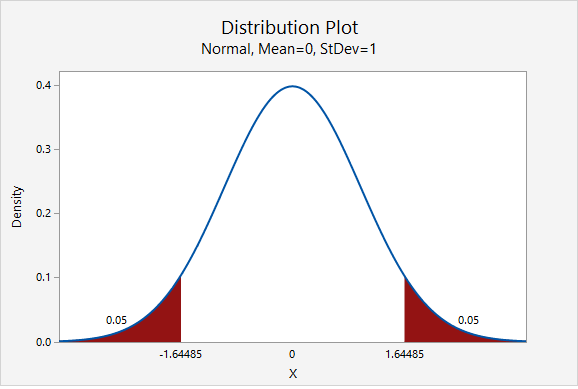# 8.2.2.3.1 - Example: Estimating IQ

8.2.2.3.1 - Example: Estimating IQ

## Example: Estimating IQ

A team of researchers wants to estimate the mean IQ of students enrolled at one prestigious university. Previous research studies have examined samples of students from other similar universities and usually find results around $$\overline{x}=120$$ and $$s=10$$. In order to construct a 90% confidence interval with a margin of error of $$\pm2$$  IQ points, what sample size should be obtained?

As shown in the probability distribution plot below, the z value associated with a 90% confidence interval is 1.645.The estimated standard deviation is given to be 10 and the desired margin of error is given to be 2.

$$n=\dfrac{z^{2}\widetilde{\sigma}^{2}}{M^{2}}=\dfrac{1.645^{2}(10^{2})}{2^{2}}=67.615$$

We round up to 68. The research team should attempt to obtain a sample of at least 68 individuals.

  Link ↥ Has Tooltip/Popover Toggleable Visibility